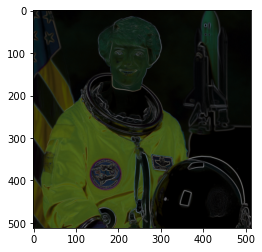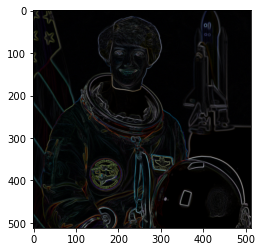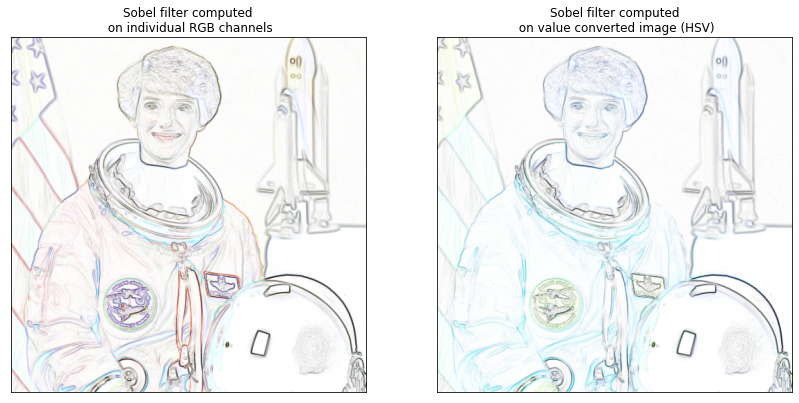There are many filters that are designed to work with gray-scale images but not with color images. To simplify the process of creating functions that can adapt to RGB images, scikit-image provides the adapt_rgb decorator.

We will demonsrate the use with the famous Sobel filter.

To actually use the adapt_rgb decorator, you have to decide how you want to adapt the RGB image for use with the gray-scale filter. There are two pre-defined handlers:

each_channel
Pass each of the RGB channels to the filter one-by-one, and stitch the results back into an RGB image.

hsv_value
Convert the RGB image to HSV and pass the value channel to the filter. The filtered result is inserted back into the HSV image and converted back to RGB.

## 3.1. Wrap filter functions with decorators¶

from skimage.color.adapt_rgb import adapt_rgb, each_channel, hsv_value
from skimage import filters

def sobel_each(image):
return filters.sobel(image)

def sobel_hsv(image):
return filters.sobel(image)


## 3.2. We can use these functions as we would normally use them, but now they work with both gray-scale and color images.¶

from skimage import data
from skimage.exposure import rescale_intensity
import matplotlib.pyplot as plt

image = data.astronaut()

image.shape

(512, 512, 3)


## 3.3. Normal Sobel filter does not work on this image¶

try:
plt.imshow(filters.sobel(image))
except ValueError as e:
print(e)## 3.4. When used with the decorator, it works¶

plt.imshow(sobel_each(image))
plt.show()## 3.5. We can take the complement and rescale the intensity¶

fig, (ax_each, ax_hsv) = plt.subplots(ncols=2, figsize=(14, 7))

# We use 1 - sobel_each(image) but this won't work if image is not normalized
ax_each.imshow(rescale_intensity(1 - sobel_each(image)))
ax_each.set_xticks([]), ax_each.set_yticks([])
ax_each.set_title("Sobel filter computed\n on individual RGB channels")

# We use 1 - sobel_hsv(image) but this won't work if image is not normalized
ax_hsv.imshow(rescale_intensity(1 - sobel_hsv(image)))
ax_hsv.set_xticks([]), ax_hsv.set_yticks([])
ax_hsv.set_title("Sobel filter computed\n on value converted image (HSV)")

Text(0.5, 1.0, 'Sobel filter computed\n on value converted image (HSV)')